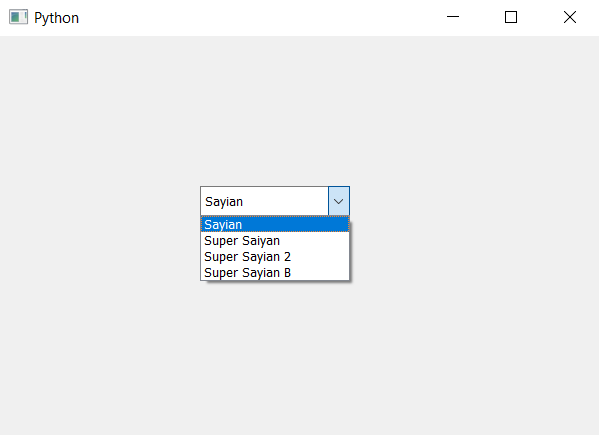# PyQt5 – Setting visible column in the model of combo box

• Last Updated : 22 Apr, 2020

In this article we will see how we can set the visible column of combo box. This is used to set the column which will be visible to the user, model column property holds the column in the model that is visible. In order to set this we use `setModelColumn` method.

Syntax : combo_box.setModelColumn(n)

Argument : It takes integer as argument i.e column index

Return : None

Below is the implementation

 `# importing libraries``from` `PyQt5.QtWidgets ``import` `*` `from` `PyQt5 ``import` `QtCore, QtGui``from` `PyQt5.QtGui ``import` `*` `from` `PyQt5.QtCore ``import` `*` `import` `sys`` ` ` ` `class` `Window(QMainWindow):`` ` `    ``def` `__init__(``self``):``        ``super``().__init__()`` ` `        ``# setting title``        ``self``.setWindowTitle(``"Python "``)`` ` `        ``# setting geometry``        ``self``.setGeometry(``100``, ``100``, ``600``, ``400``)`` ` `        ``# calling method``        ``self``.UiComponents()`` ` `        ``# showing all the widgets``        ``self``.show()`` ` `    ``# method for widgets``    ``def` `UiComponents(``self``):`` ` `        ``# creating a combo box widget``        ``self``.combo_box ``=` `QComboBox(``self``)`` ` `        ``# setting geometry of combo box``        ``self``.combo_box.setGeometry(``200``, ``150``, ``150``, ``30``)`` ` `        ``# setting visible model column ``        ``self``.combo_box.setModelColumn(``0``)``         ` `        ``# geek list``        ``geek_list ``=` `[``"Sayian"``, ``"Super Saiyan"``, ``"Super Sayian 2"``, ``"Super Sayian B"``]`` ` `        ``# making it editable``        ``self``.combo_box.setEditable(``True``)`` ` `        ``# adding list of items to combo box``        ``self``.combo_box.addItems(geek_list)`` ` ` ` `# create pyqt5 app``App ``=` `QApplication(sys.argv)`` ` `# create the instance of our Window``window ``=` `Window()`` ` `# start the app``sys.exit(App.``exec``())`

Output :My Personal Notes arrow_drop_up